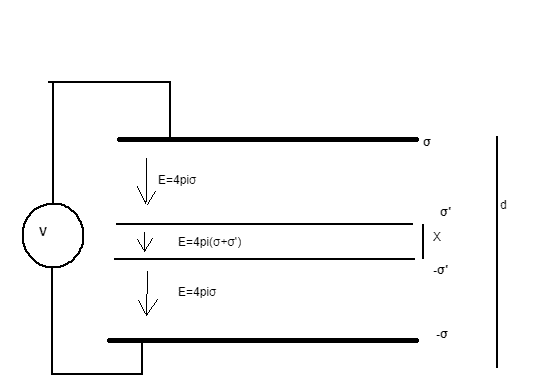# Detect if the Electric Field in a parallel plate capacitor is pointing up or down

qwereqe
Homework Statement:
Relevant Equations:
E=4πσ, V=-Ex
Assuming we have an infinite plane capacitor,where the upper plate is charged positively and the bottom layer is charged negatively. Now we know the field outside the capacitor is zero so we can't tell if the positive charge is on the upper plate or the lower plate.

But, if we place it inside another infinite plate capacitor, between its plates, and apply voltage between the external capacitor, we can't detect if it it is facing upward or downward.Using superposition, the field in the middle equals 4π(σ±σ′)4π(σ±σ′), depending of the inner plates alignment, with respect to the voltage. If the voltage is positive, we can calculate it integrating the field and get in one case:

V=4π(σd+σ′x)V=4π(σd+σ′x)

and if the inner capacitor is rotated downward we get:

V=4π(σd−σ′x)V=4π(σd−σ′x)

Where σ,dσ,d is the external capacitor charge density and distance and σ′,x is the inner capacitor charge density and distance.

So, because σ′,d,x,V are constant, we can see that the charge density on the external capacitor will change if the potential difference of the inner capacitor is aligned toward the external capacitor or not, and the force on the outer plates will be different in each case' because of the different charge density.

So, my problem is, if the field outside the inner capacitor is zero, how we can still detect its alignment? Seems, like a paradox to me.

Thanks.

Last edited:

Homework Helper
Gold Member
I am not sure I understand your post deeply, and where exactly the paradox is, but if we connect a DC voltmeter to the inner capacitor, wouldn't that tell us which plate is positive and which negative?

qwereqe
I am not sure I understand your post deeply, and where exactly the paradox is, but if we connect a DC voltmeter to the inner capacitor, wouldn't that tell us which plate is positive and which negative?
The paradox is: without access to the inner capacitor, the field outside of it is zero, so we can't tel anything about it. But if we do as I proposed, we can say if it is pointing upward or downward.

Homework Helper
Gold Member
The paradox is: without access to the inner capacitor, the field outside of it is zero, so we can't tel anything about it. But if we do as I proposed, we can say if it is pointing upward or downward.
Ok now it makes a bit more sense to me, the whole thing. But you, instead of using access to the inner capacitor, you use access to the outer capacitor which is also infinite. I mean, if we have difficulty to access the inner capacitor because it is infinite (and we going to have to use infinite connecting wires to the voltmeter e.t.c), we have the same difficulty accessing the outer capacitor.